# Tail Recursion Problems with Recursion Recursion is generally

• Slides: 19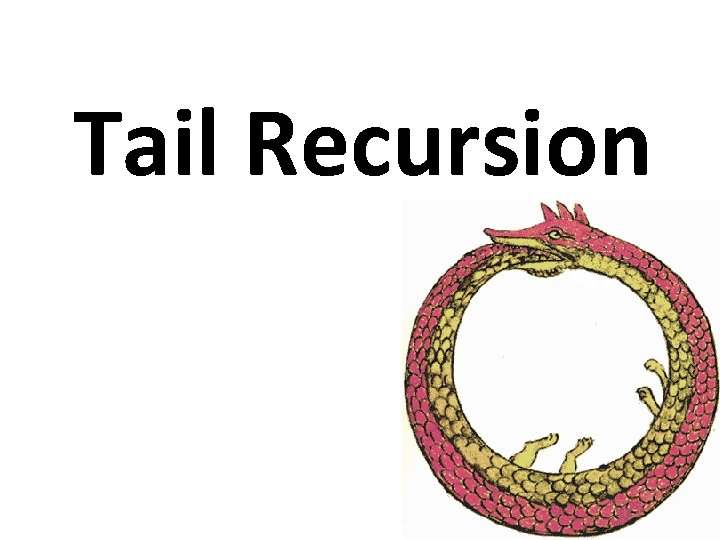Tail RecursionProblems with Recursion • Recursion is generally favored over iteration in Scheme and many other languages It’s elegant, minimal, can be implemented with regular functions and easier to analyze formally • It can also be less efficient more functional calls and stack operations (context saving and restoration) • Running out of stack space leads to failure deep recursionTail recursion is iteration • Tail recursion is a pattern of use that can be compiled or interpreted as iteration, avoiding the inefficiencies • A tail recursive function is one where every recursive call is the last thing done by the function before returning and thus produces the function’s value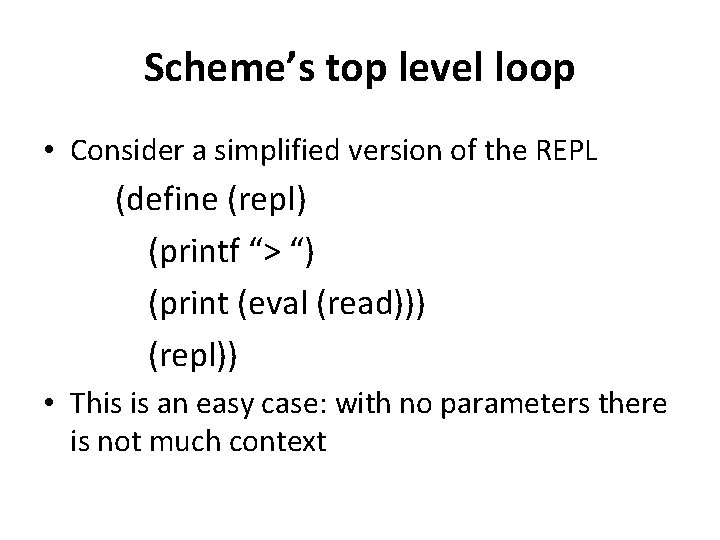Scheme’s top level loop • Consider a simplified version of the REPL (define (repl) (printf “> “) (print (eval (read))) (repl)) • This is an easy case: with no parameters there is not much context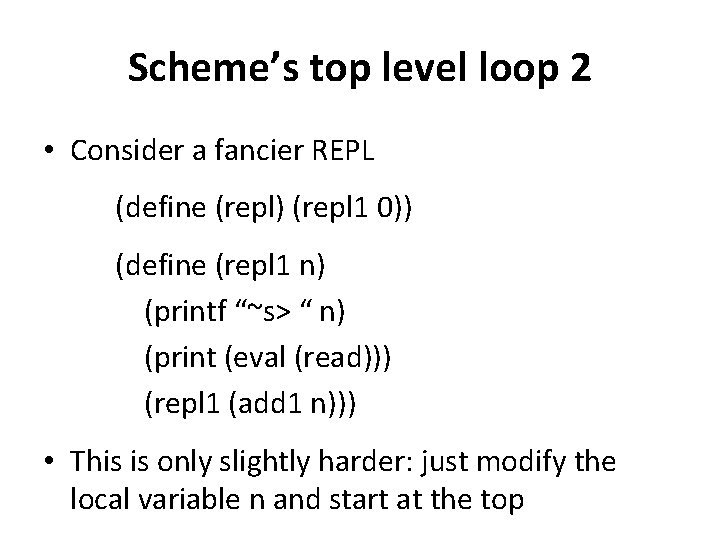Scheme’s top level loop 2 • Consider a fancier REPL (define (repl) (repl 1 0)) (define (repl 1 n) (printf “~s> “ n) (print (eval (read))) (repl 1 (add 1 n))) • This is only slightly harder: just modify the local variable n and start at the top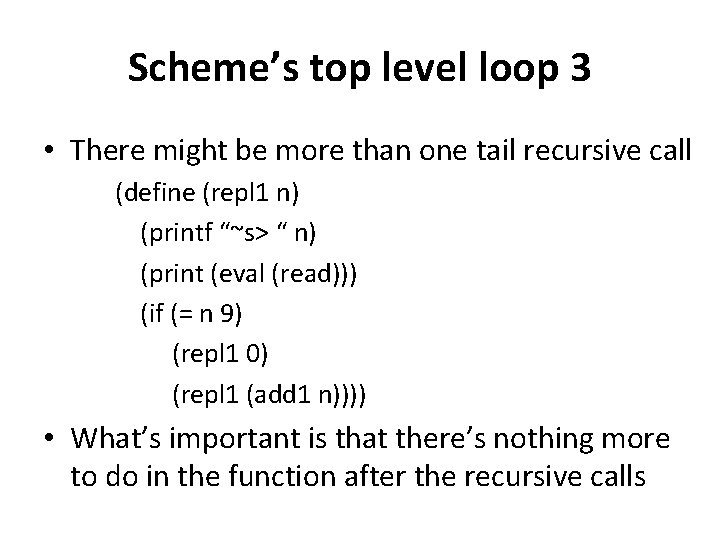Scheme’s top level loop 3 • There might be more than one tail recursive call (define (repl 1 n) (printf “~s> “ n) (print (eval (read))) (if (= n 9) (repl 1 0) (repl 1 (add 1 n)))) • What’s important is that there’s nothing more to do in the function after the recursive callsTwo skills • Distinguishing a trail recursive call fromNaïve recursive factorial (define (fact 1 n) ; ; naive recursive factorial (if (< n 1) 1 (* n (fact 1 (sub 1 n)))))Tail recursive factorial (define (fact 2 n) ; rewrite to just call the tail-recursive ; factorial with the appropriate initial values (fact 2 -helper n 1)) (define (fact 2 -helper n accumulator) ; tail recursive factorial calls itself as ; last thing to be done (if (< n 1) accumulator (fact 2 -helper (sub 1 n) (* accumulator n))))Trace shows what’s going on > (require (lib "trace. ss")) > (load "fact. ss") > (trace fact 1) > (fact 1 6) | (fact 1 5) | |(fact 1 4) | | (fact 1 3) | | |(fact 1 2) | | | (fact 1 1) | |(fact 1 0) | |1 |||1 | | |2 ||6 | |24 | 120 |720fact 2 > (trace fact 2 -helper) > (fact 2 6) |(fact 2 6) • Interpreter & compiler note the last expression to be |(fact 2 -helper 6 1) evaled & returned in fact 2|(fact 2 -helper 5 6) helper is a recursive call |(fact 2 -helper 4 30) • Instead of pushing state |(fact 2 -helper 3 120) on the sack, it reassigns |(fact 2 -helper 2 360) the local variables and jumps to beginning of the |(fact 2 -helper 1 720) procedure |(fact 2 -helper 0 720) • Thus, the recursion is |720 automatically transformed 720 into iteration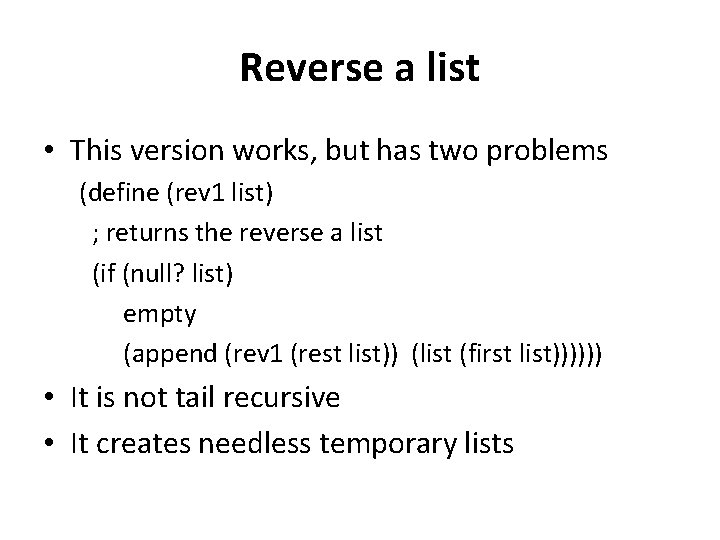Reverse a list • This version works, but has two problems (define (rev 1 list) ; returns the reverse a list (if (null? list) empty (append (rev 1 (rest list)) (list (first list)))))) • It is not tail recursive • It creates needless temporary lists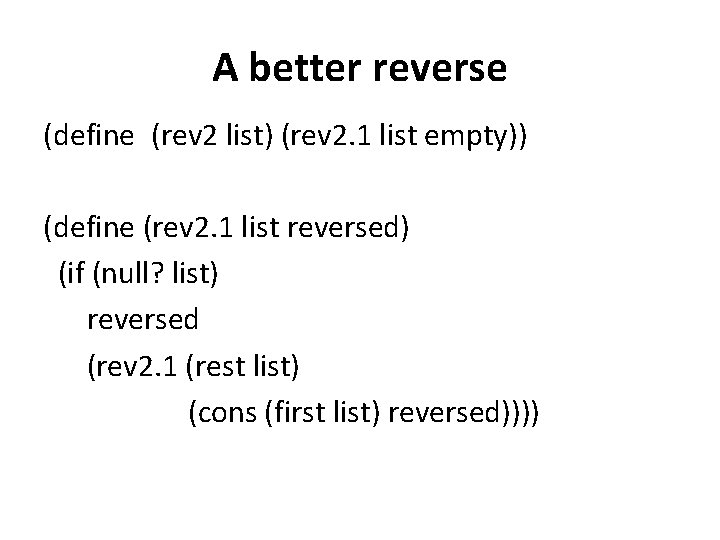A better reverse (define (rev 2 list) (rev 2. 1 list empty)) (define (rev 2. 1 list reversed) (if (null? list) reversed (rev 2. 1 (rest list) (cons (first list) reversed))))> (load "reverse. ss") > (trace rev 1 rev 2. 1) > (rev 1 '(a b c)) |(rev 1 (a b c)) | (rev 1 (b c)) | |(rev 1 (c)) | | (rev 1 ()) | | () | |(c) | (c b) |(c b a) rev 1 and rev 2 > (rev 2 '(a b c)) |(rev 2. 1 (a b c) ()) |(rev 2. 1 (b c) (a)) |(rev 2. 1 (c) (b a)) |(rev 2. 1 () (c b a)) |(c b a) >The other problem • Append copies the top level list structure of it’s first argument. • (append ‘(1 2 3) ‘(4 5 6)) creates a copy of the list (1 2 3) and changes the last cdr pointer to point to the list (4 5 6) • In reverse, each time we add a new element to the end of the list, we are (re-)copying the list.Append (two args only) (define (append list 1 list 2) (if (null? list 1) list 2 (cons (first list 1) (append (rest list 1) list 2))))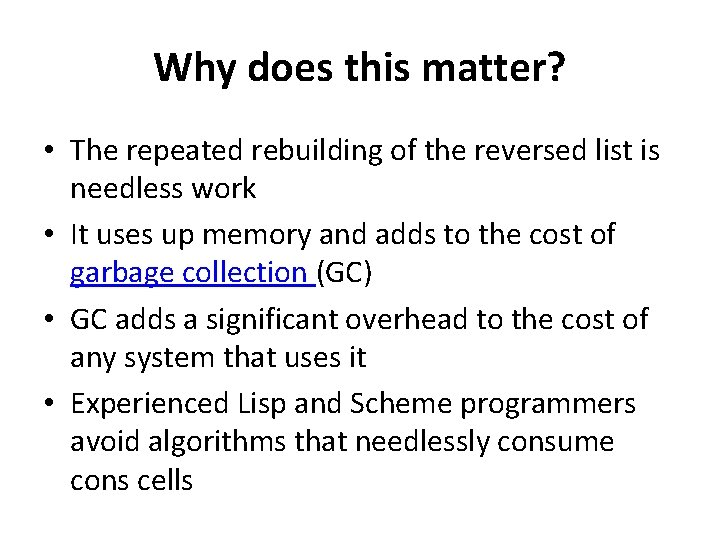Why does this matter? • The repeated rebuilding of the reversed list is needless work • It uses up memory and adds to the cost of garbage collection (GC) • GC adds a significant overhead to the cost of any system that uses it • Experienced Lisp and Scheme programmers avoid algorithms that needlessly consume cons cellsFibonacci (define (fib n) ; ; naive recurseive fibonacci function (if (< n 3) 1 (+ (fib (- n 1)) (fib (- n 2))))) Run time for fib(n) ≅ O(2 n)Fibonacci (define (fib 2 n) (if (< n 3) 1 (fib-tr 3 n 1 1))) (define (fib-tr n stop fib. n-2 fib. n-1 ) (if (= n stop) (+ fib. n-1 fib. n-2) (fib-tr (+ 1 n) stop fib. n-1 (+ fib. n-1 fib. n-2)))) Run time for fib(n) ≅ O(n)